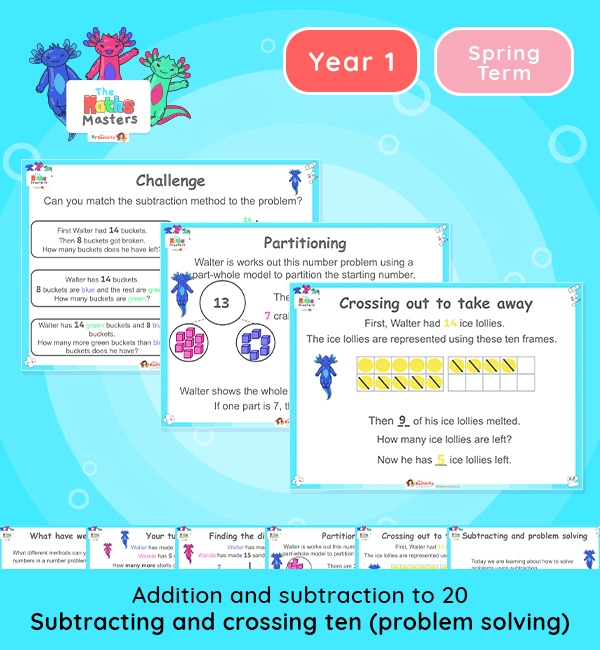Year 1 | Subtracting and Crossing 10 (Problem Solving) Lesson PresentationWhite Rose Year 1 addition and subtraction lesson plans

Aligned with the White Rose Maths scheme of work, this Year 1 | Subtracting and Crossing 10 (Problem Solving) Lesson Presentation is fully editable, and is designed for the Year 1 maths curriculum covering the following maths objectives for the autumn term:

Topic: Addition and subtraction to 20

This lesson focuses on subtracting numbers within 20 and crossing ten. It focuses on problem solving and subtracting to find the difference using partitioning and other methods of subtraction.

White Rose Maths Small Steps: Subtraction crossing ten (2)

NC Links: read, write and interpret mathematical statements involving addition, subtraction and equals signs. *Add and subtract one-digit and two-digit numbers to 20, including zero. *Solve one step problems that involve addition and subtraction using concrete objects and pictorial representations , and missing number problems.

TAF Statements: Working towards: Add and subtract one digit number explaining their method verbally, in pictures, or using apparatus.

Working At: Recall all the number bonds to and within 10 and use these to reason with and calculate bonds to and within 20, recognising other associated additive relationships.

Greater Depth – Use reasoning about number and relationships to solve more complex problems and explain their thinking.

Ready-to-progress criteria:  1AS-2 Read, write and interpret equations containing addition, subtraction and equals symbols and relate additive expressions and equations to real-life contexts.

Previous experience: Devise and record number stories, using pictures, numbers and symbols.